Japanese

#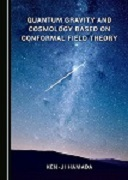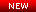"Quantum Gravity and Cosmology Based on Conformal Field Theory"
By Ken-ji Hamada, Cambridge Scholars Publishing, 2018
For details, see Books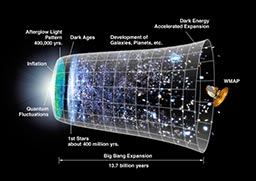The discovery of the cosmic microwave background radiation (CMB) by Penzias and Wilson has proven that the universe was once a melting pot of high energy reactions at its early stage, and since then it has been cooling as it expands. Valuable information on physical processes taking place in the expanding universe has been recorded in the CMB as tiny anisotropies. Angular power spectra of the anisotropies, recently observed by the Cosmic Background Explorer (COBE) and the Wilkinson Microwave Anisotropy Probe (WMAP), are, roughly speaking, projection of the history for the period between its birth to the present. It gives us a amazing hope that if we believe the idea of inflationary universe describing a extremely rapid expansion without global thermalization, the long-distance correlation in observed anisotropies can provide the information about dynamics of the period before the universe grew to the Planck scale. We are now on the stage of revealing and verifying the quantum aspect of universe.

After passing many theoretical as well as experimental tests, the general theory of relativity has been established as the fundamental theory of gravity capable of describing the evolution of universe. Many questions in the mysteries of universe have arose expecting that the theory can give answers, such as, how the universe is created, why it is expanding, how it will evolve in the future, and so on. In order to answer these questions, we need to know the initial condition of the universe that would be set at the Planck time. However, it is the period when quantum mechanics rules the law of universe, and it is beyond the power of the classical general relativity. If we wish to apply the Einstein theory for the Planck scale phenomena, it has fatal difficulties, such as the black-hole singularity and divergences in the canonical quantization procedure. Existence of these problems makes us wonder whether the Planck scale is the upper limit of the energy beyond which nature cannot be described in terms of physical laws. It is our ultimate task to construct the quantum theory which breaks the wall of Planck scale and creates a new frontier.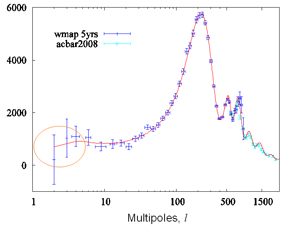Renormalizable quantum theory of gravity developed on the basis of the conformal gravity in four dimensions provides an evolutional scenario of the universe which starts from quantum states with conformal invariance, passing through the inflationary era, and then makes transition to the conventional Einstein space-time without adding any artificial field by hands. In this theory the metric tensor field is written as a product of the conformal factor and the traceless tensor, and the conformal mode is treated non-perturbatively while the traceless tensor field is handled as a perturbation. The renormalized coupling of the traceless tensor mode indicates the asymptotic freedom. It implies that at very high energies beyond the Planck mass scale, quantum fluctuations of the conformal mode are dominated as a consequence of the background-metric independence, and space-time is non-singular. This nature is called ``asymptotic background freedom'' to distinguish from the normal asymptotic freedom. The power-law spectrum obtained from the two point correlation function of scalar curvature shows a significant character of the conformally invariant field theory which is expected to be observed cosmologically.

The asymptotic background freedom also implies that there exists a dynamical scale where the effective coupling of traceless tensor mode diverges, and the conformal symmetry loses its validity. About this point the universe is expected to make a transition from the quantum spacetime to the classical Einstein spacetime, and fluctuations of the conformal mode would be frozen to the classical background. Such a dynamical scale is given by the order of 10^17GeV below the Planck scale. The sharp fall off of the angular power spectra at low multipole components of anisotropies in the CMB is expected to be an appearance of dynamical scale.

### 《Asymptotically Background-Free Quantum Gravity》

The Einstein theory defined by the Ricci scalar is known to be not renormalizable, but it does not implies that diffeomorphism invariance conflicts with renormalization theory. Renormalizable quantum gravity presented here is defined by the following action determined by imposing the conditions of diffeomorphism invariance and Wess-Zumino integrability:The first term is the Weyl action and "t" is the dimensionless coupling constant with negative beta function, which controls dynamics of the traceless tensor field. On the other hand, the conformal factor in the metric field is treated non-perturbatively without introducing its own coupling constant. The path integral over the metric field is described as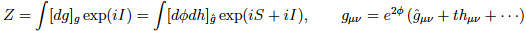Here, S is the Wess-Zumino action for conformal anomalies induced from the path integral measure. It is a Jacobian required to preserve diffeomorphism invariance when rewriting the path integral using the practical measure defined on the background metric. Especially, the action S that appears at the ultraviolet (UV) fixed point of t=0 is called the Riegert-Wess-Zumino action, which is given byAt the UV fixed point, the theory is described as a certain conformal field theory (CFT). Exact conformal symmetry comes out as a part of quantum diffeomorphism invariance, realized by taking the sum over all possible conformally flat configurations. The background-metric independence is then represented as a gauge equivalency under conformal transformations, called BRST conformal symmetry. This is nothing but a realization of the ``asymptotic safety" program proposed by S. Weinberg in 1970s, as a condition for quantum gravity to be well-defined.

The BRST CFT represents a strong coupling phase in which gravity is fully fluctuating. On the other hand, the coupling t, which measures a degree of devitation from the CFT, describes another strong couping phase. With the increase of it, spacetime will make a transition from quantum gravity phase to classical Einstein phase, as mentioned above.

### 《Novel Interpretation of Minimal Length》

The inverse of the dynamical energy scale mentioned above gives the correlation length of quantum gravity. If shorter than this length, we cannot measure the distance, which is due to the background-metric independence where the concept of time and space is lost. Thus, it represents the minimal length of space-time we can measure physically. In this sense, space-time is practically quantized by this length unit without descretizing it. The energy of excitations in quantum gravity would be then given by the dynamical scale. If there is a stable excitation, it becomes a candidate for dark matter.

## PROFILE

• Name: Ken-ji HAMADA
• Affiliation: Institute of Particle and Nuclear Studies, KEK, Tsukuba, Japan
• Research Interests: Renormalizable Quantum Gravity, Conformal Field Theory and Cosmology

## RESEARCH ACTIVITIES

### Books"Quantum Gravity and Cosmology Based on Conformal Field Theory"
By Ken-ji Hamada (Cambridge Scholars Publishing, 2018) [
View Extract] [Errata]
 Table of Contents Chapter 1 Introduction Chapter 2 Conformal Field Theory in Minkowski Space Chapter 3 Conformal Field Theory in Euclidean Space Chapter 4 Basis of Two-Dimensional Conformal Field Theory Chapter 5 Conformal Anomaly and Wess-Zumino Action Chapter 6 Two-Dimensional Quantum Gravity Chapter 7 Background-Free Quantum Gravity Chapter 8 Physical States of Quantum Gravity Chapter 9 Gravitational Counterterms and Conformal Anomalies Chapter 10 Renormalization in Quantum Gravity Chapter 11 The Universe in Einstein Gravity Chapter 12 Quantum Gravity Cosmology Chapter 13 Cosmological Perturbation Theory Chapter 14 From Quantum Gravity to CMB Appendix A, B, C, D, E Bibliography

[Abstract] In this book, I will describe a renormalizable quantum gravity formulated with incorporating a new technique based on conformal field theory which recently has made prominent progress. Conformal invariance here appears as a gauge symmetry that gives a key property of quantum gravity known as the background-metric independence. Due to the presence of this symmetry, the theory becomes free from the problem of spacetime singularity, and thus from the information loss problem, namely the ghost problem as well. Furthermore, I will give a new scenario of the universe that evolves from quantum gravity world to the current classical world through the spacetime phase transition, including inflation driven by quantum gravity effects only.

This book also includes descriptions of recent developments in conformal field theory, renormalization theory in curved space, and conformal anomalies related thereto, almost of which do not found in other books. In addition, it includes review on evolution equations of the universe that is the foundation of modern cosmology necessary to understand results of the CMB experiments such as WMAP. Furthermore, it will be briefly shown that there is a noticeable relationship between the quantum gravity and a random lattice model that is based on the dynamical triangulation method known as another description of the background free property. I would like to describe these topics by taking enough pages as a latest advanced textbook for leading to this new area of quantum field theory that developed mainly since the beginning of this century.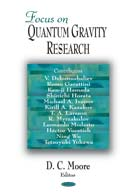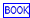Background Free Quantum Gravity and Cosmology
K. Hamada, S. Horata and T. Yukawa, ``Focus on Quantum Gravity Research" (Nova Science Publisher, NY, 2006), Chap. 1.
 Contents Section 1 Introduction Section 2 Renormalizable Quantum Gravity 2.1 Brief Summary of The Model 2.2 Conformal Invariance and Physical States 2.3 Renormalization Section 3 Numerical Quantum Gravity Section 4 Cosmology Section 5 Conclusion

[Abstract] Tracing the history of the universe, spacetime in its early epoch would be totally fluctuating quantum mechanically, and there would be no classical spacetime to describe dynamics. The models of quantum gravity we are going to discuss are aimed to describe such states with background-metric independence and transitions to the conventional spacetime dynamics.

The first model we choose is a renormalizable theory of gravity developed on the basis of the conformal gravity in four dimensions. In this theory the metric tensor field is written as a product of the conformal factor and the traceless tensor, and the conformal mode is treated non-perturbatively while the traceless tensor field is handled as a perturbation. The renormalized coupling of the traceless mode indicates the asymptotic freedom. It implies that at very high energies beyond the Planck mass scale, quantum fluctuations of the conformal field are dominated as a consequence of the background-metric independence. The second model we plan to discuss is a numerical method for quantizing gravity carried on the simplicial spacetime manifold. The theory treats dynamics non-perturbatively within the limited space allowed in a cluster of computers. It is significant that these two theories seem to be in the same universality class so far as the critical exponents are compared. It means that a conformal invariant spacetime can be mapped to a simplicial manifold with varying connectivity of simplices.

The asymptotic freedom also implies that there exists a dynamical scale where the effective coupling of traceless mode diverges, and the conformal symmetry loses its validity. About this point the universe is expected to make a transition from the quantum spacetime to the classical Einstein spacetime, and fluctuations of the conformal field would be frozen to the classical background. The primordial power spectrum obtained from the two point correlation function of scalar curvature shows a significant character of the theory which is expected to be observed cosmologically. The sharp fall off of the angular power spectra at low multipole components of anisotropies in the cosmic microwave background is a topic we wish to discuss.

### Research papers (selected)

Quantum Gravity Cosmology

1. From CFT Spectra to CMB Multipoles in Quantum Gravity Cosmology,
K. Hamada, S. Horata and T. Yukawa, Phys. Rev. D81 (2010) 083533, arXiv:0908.0192[astro-ph].
2. Analyzing WMAP Observation by Quantum Gravity,
K. Hamada, S. Horata, N. Sugiyama and T. Yukawa, Prog. Theor. Phys. 119 (2008) 253, arXiv:0705.3490[astro-ph].
3. Space-time Evolution and CMB Anisotropies from Quantum Gravity,
K. Hamada, S. Horata and T. Yukawa, Phys. Rev. D74 (2006) 123502, astro-ph/0607586.
4. CMB Anisotropies Reveal Quantized Gravity,
K. Hamada and T. Yukawa, Mod. Phys. Lett. A20 (2005) 509, astro-ph/0401070.
5. Baryogenesis by Quantum Gravity,
K. Hamada, A. Minamizaki and A. Sugamoto, Mod. Phys. Lett. A23 (2008) 237, arXiv:0708.2127 [astro-ph].

Quantum Diffeomorphism and Conformal Field Theory

1. BRST Conformal Symmetry as A Background-Free Nature of Quantum Gravity,
2. BRST Analysis of Physical Fields and States for 4D Quantum Gravity on R x S^3,
K. Hamada, Phys. Rev. D86 (2012) 124006, arXiv:1202.4538[hep-th].
3. BRST Invariant Higher Derivative Operators in 4D Quantum Gravity based on CFT,
K. Hamada, Phys. Rev. D85 (2012) 124036, arXiv:1203.4894[hep-th].
4. Background Free Quantum Gravity based on Conformal Gravity and Conformal Field Theory on M^4,
K. Hamada, Phys. Rev. D85 (2012) 024028, arXiv:1109.6109[hep-th].
5. Conformal Field Theory on R x S^3 from Quantized Gravity,
K. Hamada, Int. J. Mod. Phys. A24 (2009) 3073--3110, arXiv:0811.1647[hep-th].
6. Building Blocks of Physical States in a Non-Critical 3-Brane on R x S^3,
K. Hamada, Int. J. Mod. Phys. A20 (2005) 5353--5398, hep-th/0402136.
7. Conformal Algebra and Physical States in a Non-critical 3-brane on R x S^3,
K. Hamada and S. Horata, Prog. Theor. Phys. 110 (2003) 1169, hep-th/0307008.

Renormalization in Quantum Gravity

1. Physical Cosmological Constant in Asymptotically Background Free Quantum Gravity,
K. Hamada and M. Matsuda, Phys. Rev. D96 (2017) 026010, arXiv:1704.03962[hep-th].
2. Two-Loop Quantum Gravity Corrections to Cosmological Constant in Landau Gauge,
K. Hamada and M. Matsuda, Phys. Rev. D93 (2016) 064051, arXiv:1511.09161[hep-th].
3. RG Analysis for Quantum Gravity with A Single Dimensionless Coupling,
K. Hamada, Phys. Rev. D90 (2014) 084038, arXiv:1407.4532[hep-th].
4. Determination of Gravitational Counterterms Near Four Dimensions from RG Equations,
K. Hamada, Phys. Rev. D89 (2014) 104063, arXiv:1403.4354[hep-th].
5. Resummation and Higher Order Renormalization in 4D Quantum Gravity,
K. Hamada, Prog. Theor. Phys. 108 (2002) 399, hep-th/0203250.
6. Background-metric Independent Formulation of 4D Quantum Gravity,
K. Hamada and F. Sugino, Nucl. Phys. B553 (1999) 283, hep-th/9810095.

Two Dimensional Quantum Gravity

1. Quantum Theory of Dilaton Gravity in 1 + 1 Dimensions,
K. Hamada, Phys. Lett. B300 (1993) 322, hep-th/9206071.
2. Physical States, Factorization and Non-linear Structures in Two Dimensional Quantum Gravity,
K. Hamada, Nucl. Phys. B413 (1994) 278, hep-th/9309094.
3. Ward Identities of W_infinity Symmetry in Liouville Theory coupled to c_M < 1 Matter,
K. Hamada, Phys. Lett. B324 (1994) 141, hep-th/9311047.
4. Non-linear Structures in Non-critical NSR String,
K. Hamada and H. Ishikawa, Commum. Math. Phys. 176 (1996) 401, hep-th/9405130.

Others

Publication list by INSPIRE